Nearpod version available

Grade 9-12
,
Lesson

# Analyzing the Business Cycle with Piecewise Functions

Time: 80 mins,
Updated: March 24 2021,
Author: Beth Rempe

### Objective

Students will be able to:

• Explain how changes in economic conditions affect real GDP.
• Generalize the business cycle as a piecewise function.
• Discuss the business cycle in terms of a function.
• Find the extrema of a function and use it to compare economic conditions.

Prior Knowledge

Students should be able to:

• Write a linear equation in slope-intercept form given two points.
• Use the vertex and standard form of a quadratic equation.
• Evaluate and solve equations.

### Concepts

In this personal finance lesson, students investigate the business cycle by interpreting the business cycle as a piecewise function.

### Procedure

In the past 100 years, moments in history like the Great Depression or more recently the housing boom and crash has heightened our awareness of the peaks and troughs in our economy. Economically speaking, these highs (peaks) and lows (troughs) are telling of the Real GDP which many economists use to measure the successfulness of a countries economy and well-being. Students can use their knowledge of mathematics in using the vertex of a quadratic function, applying properties of linear equations, and analyzing non-linear functions to quantitatively describe and compare different cycles in the economy. Students can then compare present situations with those in the past.

1. Introduce the concept of gross domestic product or GDP by showing the clip https://www.stlouisfed.org/education/economic-lowdown-video-series/episode-7-gross-domestic-product .
2. Display Slide1 as a prompt for discussion. Ask students to work with a partner to answer the questions. Review answers showing Slide 2. In the discussion, students should take away that GDP is the market value of all final goods and services produced in a country in a calendar year and is used to determine the size of the economy at a point in time. Real GDP is GDP measured in dollars of constant purchasing power. The measure is obtained by adjusting nominal GDP (GDP measured in current prices) by an appropriate price index, usually the implicit price deflator. Real GDP can show the growth of an economy over time and ideally should be higher than the previous years. Other words and their meaning should be understood:
• Market value: the price paid for a good or service in the marketplace.
• Final goods and services are goods and services produced for consumers or end users.
• Give an example of when people purchase flour at the store to produce cookies or bread at home it is counted as part of real GDP but when flour is sold to bakeries that produce the baked goods for retail, the baked goods are counted as part of real GDP but the flour itself is not.
• Intermediate goods are not counted as part of real GDP but go into the production of a good or service. The value of the flour used to produce the bread at the bakery will be reflected in price of the final product when it is sold to the end user. In the example above, flour when used by the bakery, is an intermediate good.
3. Have students use what they have learned in the video to classify examples of goods or services that would or would not be included in the calculation of real GDP.
4. Divide the class into two even groups (have a member of the smaller group go twice if there are an odd number of students). Have them line up in two rows in front of the board. Give each student a copy of Activity 1. Distribute a paddle to the first student in each row. Instruct students that a series of slides will be displayed. For each slide the student holding the paddle must decide if the good or service would or would not be included in the calculation of real GDP, then display the appropriate side of the paddle, and then return to their seat. For each correct paddle answer, the team gets a point. The team with the most points wins.
5. Display Slides 3-17, one at a time. After each team member holds up the paddle, ask the class what the correct answer is. Have students record the correct answer on Activity 2.
Answers for the scenarios:

 Scenario 1 Eating out at your favorite local restaurant Real GDP Scenario 2 Service station attendant changes your flat tire Real GDP Scenario 3 Dad helping his child change a flat tire NOT Scenario 4 An Italian vacationing in New York City Real GDP Scenario 5 An American vacationing in Italy NOT Scenario 6 Buying a bracelet while vacationing in Japan NOT Scenario 7 Buying a chair from a factory in the US Real GDP Scenario 8 Bread sold to a family in Kansas Real GDP Scenario 9 The bread sold to McDonald’s to use for hamburgers in Kansas NOT Scenario 10 Buying your prom dress Real GDP Scenario 11 Catering for your prom Real GDP Scenario 12 Your first brand new car Real GDP Scenario 13 Tires installed on a car at the factory NOT Scenario 14 Going to the movie theater Real GDP Scenario 15 Getting snacks at the movie theater Real GDP

6. Ask students to work with a partner and record on Activity 2 why a good or service would be included or not included in real GDP. (See Activity 2 Answer Key. Students should be able to explain that for something to be included in real GDP it must be purchased by the end user and be produced in the United States. Nonmarket activities such as baking a cake at home are not included. Numbers 1, 2, 4, 7, 8, 10, 11, 12, 14 and 15 are goods and services purchased by the end user. Numbers 5 and 6 are purchased outside the United States. Numbers 9 and 13 are intermediate goods. Number 3 is a nonmarket activity.)
7. Tell students that economic variables that can affect real GDP include:
• Business investment—When the economy is growing, businesses tend to expand and spend more on new plants and equipment, produce more products, and hire more workers. When the economy is contracting, businesses spend less on expansion, may reduce production, and lay off workers.
• Consumer expectations—If consumers expect the economy to expand resulting in more jobs and increases in income, they will more likely buy more goods and services. If consumers expect a contraction, they may save more and spend less.
• Interest rates and credit—When interest rates are low, businesses tend to borrow to make new investments and consumers will use more credit to purchase goods and services. The reverse is true when interest rates rise.
• External Shocks—External shocks include things such as droughts and disruption of the oil supply. These have negative impacts on the growth of the economy.
8. Display Slide 18. For each headline ask the class how the situation in the headline would affect real GDP. Would it increase or decrease? (Headlines 1, 4, and 5 will result in declines in real GDP and headlines 2, 3, and 6 increases in real GDP.)
9. Tell students that these fluctuations in real GDP can be shown on a graph. Display Slide 19. Explain this picture is called a business cycle. A business cycle is the fluctuating levels of economic activity in an economy over a period of time measured from the beginning of one recession to the beginning of the next, usually measured by real gross domestic product.
10. Ask the students what function would best represent the graph of the business cycle shown in Slide 19. (Answers may vary from linear, quadratic, cubic, trigonometric; the best answer would be a piecewise function).
11. Show Clip http://www.econedlink.org/interactives/index.php?iid=200&type=educator
12. After the video clip, briefly discuss the following points to check for understanding:
1. What was represented on the y-axis in the video clip? (Output or real GDP)
2. What was represented on the x-axis in the video clip? (Time)
3. What is a peak? (In a business cycle, a high level of output and employment, normally followed by a decline in output and employment.)
4. What is the trough? (In a business cycle, a low level of output and employment, normally followed by a rise in output and employment.)
5. What are some types of functions you saw in the video clip? (Quadratic functions)
6. Can there be any other types of functions? (Yes; growth or decay can be linear, cubic, quartic, exponential, etc.)
7. What is it called when we piece together many different types of functions? (A piecewise function)
13. Explain to students that parts of a business cycle can be compared to quadratic parabolic functions and other parts of the business cycle can be compared to linear functions. This is why looking at the business cycle as a piecewise function can help us better analyze specific sections more accurately. Additionally, in the business cycle, the peaks and the troughs are crucial turning points for the economy. These peaks and troughs are the vertices on the parabolas.
14. Remind students that in real data, the x-axis displays the time in years and the real GDP is represented on the y-axis. Students should already be familiar with (x, f(x)) notation. Remind students that the standard form of a line is f(x) = mx + b or y = mx + b and that the standard form of a quadratic function is f(x) = ax2 +bx +c or y = ax2 +bx +c and when it is in the form f(x) = a(x – h)2 +k it is the vertex form where the vertex is represented by (h, k).
15. Use Slides 20-24 to discuss how the function may at first look difficult to write a rule for but can be broken into pieces to simplify talking about the function quantitatively. For each piece of the function, work through the mathematics of how to write the function over that domain.
1. Display Slide 20. Ask students what type of functions they see.
(Quadratic and linear)
2. Display Slide 21. Notice that from 1< x < 4.5 the function resembles a quadratic function. Use the vertex formula from a quadratic function y = a(x – h)2 + k along with the point (2, 30) and vertex (3, 40) as seen on the graph to solve for a.
The point represents (x, y) and the vertex represents the values for (h, k).

y = a(x – h)2 + k
30 = a(2 – 3)2 + 40
30 = a(-1)2 + 40
30 = 1a + 40
-10 = 1a
-10 = a

With the a just found and the given vertex, write the quadratic function for all points on the curve.

y = -10(x – 3)2 + 40

The equation could also be simplified to be represented in standard form.

y = -10(x2 – 3)(x – 3) + 40
y = -10(x2 – 3x – 3x + 9) + 40
y = -10(x2 – 6x + 9) + 40
y = -10x2 + 60x – 90 + 40
y = -10x2 + 60x – 50

3. Display Slide 22. Repeat same process as in step b except the vertex this time is (7, 5) and another point on the curve is (5, 15). Notice that from 4.5 < x < 11 the function resembles a quadratic function. Use the vertex formula from a quadratic function y = a(x – h)2 + k along with the point (5, 15) and vertex (7, 5) as seen on the graph to solve for a. The point represents (x, y) and the vertex represents the values for (h, k).With the a just found and the given vertex, write the quadratic function for all points on the curve.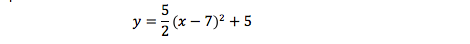The equation could also be simplified to be represented in standard form.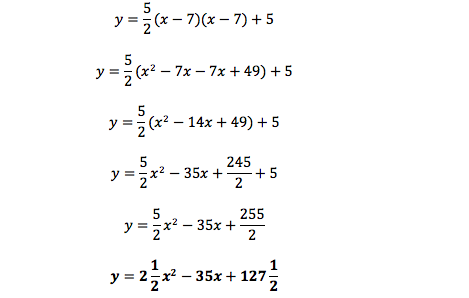4. Display Slide 23. Notice that from 11 < x < 14 the function resembles a linear function. Use two points on the line to write an equation in slope-intercept form of y = mx + b.Given the points (11, 30) and (14, 70) on the line, find slope:Use one of the points and the found slope to solve for the y-intercept (b).Write the linear function for all points on the line.5. Display Slide 24. Now you can put all three parts of the function you found together in piecewise notation. You just have to write each function over the interval that it appears. Therefore,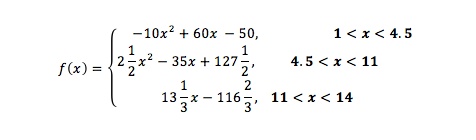16. Discuss with the students that the a coefficient controls how wide or skinny the quadratic function opens. The larger the number, the skinnier the curve. When a is positive, the function opens up, and when the a is negative the function opens down.
17. Hand out Activity 3, one copy per student. Display Slide 25, Business Cycle Example, and ask students to work on analyzing the first graph by breaking it down into the shapes of functions they are familiar with which is linear and quadratic. These pieces when talked about together are called piecewise functions. Students should be able to create the piecewise function based on the same process demonstrated to them in Step 15. (Answers are as follows: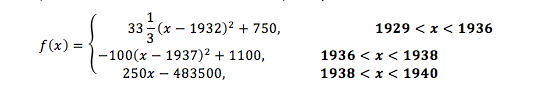18. Ask the students the following questions about the function they just wrote, the piecewise equation, as it pertains to real GDP:
1. What does a quadratic function with a negative a coefficient represent in terms of real GDP? (It stands for a function opening downwards which means a recession is happening after the vertex.)
2. Is the vertex on the function with a negative a coefficient a peak or a trough? (Peak)
3. What does a quadratic function with a positive a coefficient represent in terms of real GDP? (It stands for a function opening upwards which means a recovery is happening after the vertex.)
4. Is the vertex on the function with a positive a coefficient a peak or a trough? (Trough)
5. If the a coefficient on the quadratic component of the piecewise function is larger than the previous a coefficient on a previous quadratic component of the piecewise function, what does that mean in terms of real GDP? (It means the recovery or recession is happening quicker than before.)
19. Display Slide 26, Business Cycle from FRED®. Ask students the similarities between the two graphs. (Both have similar dips and similar size business cycles and numerically about the same.) Ask students if they can see any differences. (Answers may vary but no big difference between them.)
20. Explain that the picture they are looking at is the actual data of the United States real GDP from 1929-1940. Ask the students what they know about the period they are looking at. (Great Depression, war, etc.)
21. Have the students finish Activity 3 by analyzing the second graph in the same manner as before. Pair their work with Slide 27, Business Cycle Example. (Students should answer as follows: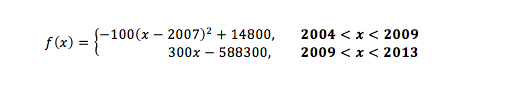22. Show Slide 28, Business Cycle from FRED®, that shows the real GDP data from the last 13 years. Instruct students to compare the pictures of this graph, (slide 28), and the earlier graph (slide 26) as well as the functions they came up with. Ask the students the following:
1. What are some differences between the first and the second graph? (Answers may vary but one answer is the numbers on the y-axis or the graph of real GDP are a lot larger in slide 28 than slide 26.)
2. What are some similarities? (The second half of the graph in slide 26 looks just like the graph in slide 28; also after the upside down parabola on each graph it is followed by a steep positive sloping line.)
3. Are there any a coefficients that are the same from graph one and graph two? (Both upside quadratic functions on the two graphs have coefficients of -100.)
4. What happened after the piece of the function with the a coefficient of -100 in both graphs? (The function had a steep positive linear growth both of relatively close slopes numerically.)
5. What was happening in the United States during the time frame of the second graph (slide 28)? Ask if anyone knows what caused the peak and recession? (Answers may vary; one response may relate to the housing market – the housing boom and crash had a big role in this rapid increase and then significant drop).
23. Explain that the two graphs represent two different periods of recession in the history of the United States’ economy. Yet, they have very similar functions that represent them. In fact, the recession had the same coefficient on the quadratic functions which means the real GDP reacted the exact same for both time periods. Additionally, recovery for both was in almost the same manner as seen by the relative similar slopes on the linear function.

1. Recap that the peaks and troughs of a business cycle are found by the vertex of quadratic functions. The vertex of that function is either a minimum or maximum. It is a minimum (a trough in a business cycle) if it is a positive function that opens upwards; it is a maximum (a peak in a business cycle) if it is a negative function that opens downwards. These functions can help see how quick or slow the recession or recovery is in the economy.
2. Display Slide 29. This is the real GDP of the United States from 1929-2010. Although the business cycle has its ups and downs, the overall growth of real GDP is a sign of a successful economy for America.
3. The patterns seen in the 85 years shown in slide 29 demonstrate that history repeats itself. The economy as measured by real GDP is just one of the many patterns that can be explored with math.

### Assessment

1. Given the following equations,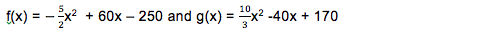describe in words what these functions look like and give a scenario in the economy that matches what they represent.(f(x) is a downward facing quadratic function whereas g(x) is an upward facing quadratic function; this can be seen by the negative and positive coefficient on the first term of each function respectively. A recession of any sort due to decreased production could be a scenario where f(x) is seen; while, g(x) could be seen as new businesses emerge.)
2. Which of the following would be calculated in GDP?
1. Sally volunteers to walk the neighbor’s dog.
2. US citizen buys souvenirs in Spain.
3. Grandparent buys a gift for grandchild.
4. Child makes a birthday cake for her brother.

Why are business cycles usually quadratic and not linear? (A typical pattern for a business cycle is that an economy begins to expand and then spending and production build upon themselves to accelerate the expansion. Some change occurs to slow the growth. A leveling off occurs and the economy may begin to shrink. That shrinkage may accelerate and then begin to slow as the economy approaches a trough.)

3. From the following graph, how many pieces would you break the function into in order to write a piecewise function and describe in words what each piece’s function would be?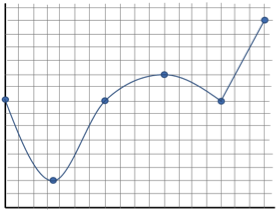ANSWER

Three pieces; the first piece would be an upward facing quadratic function, the second piece would be a wider downward facing quadratic function, and the third piece would be a positively sloped linear function.